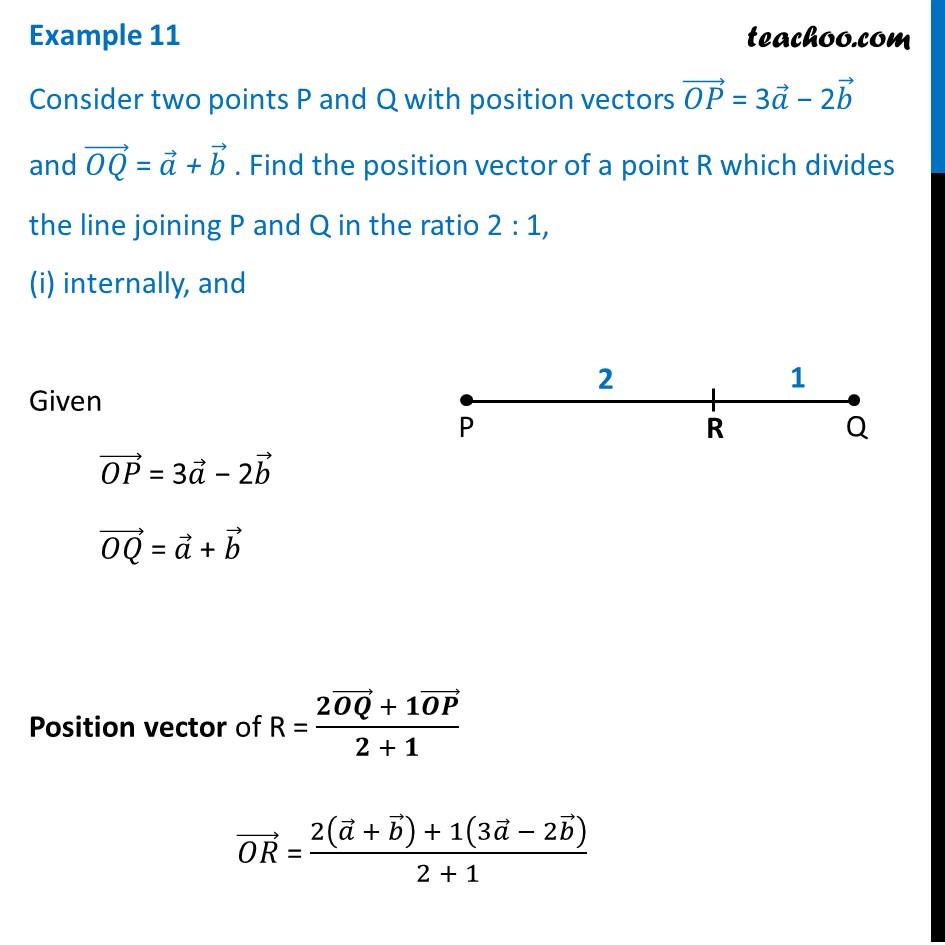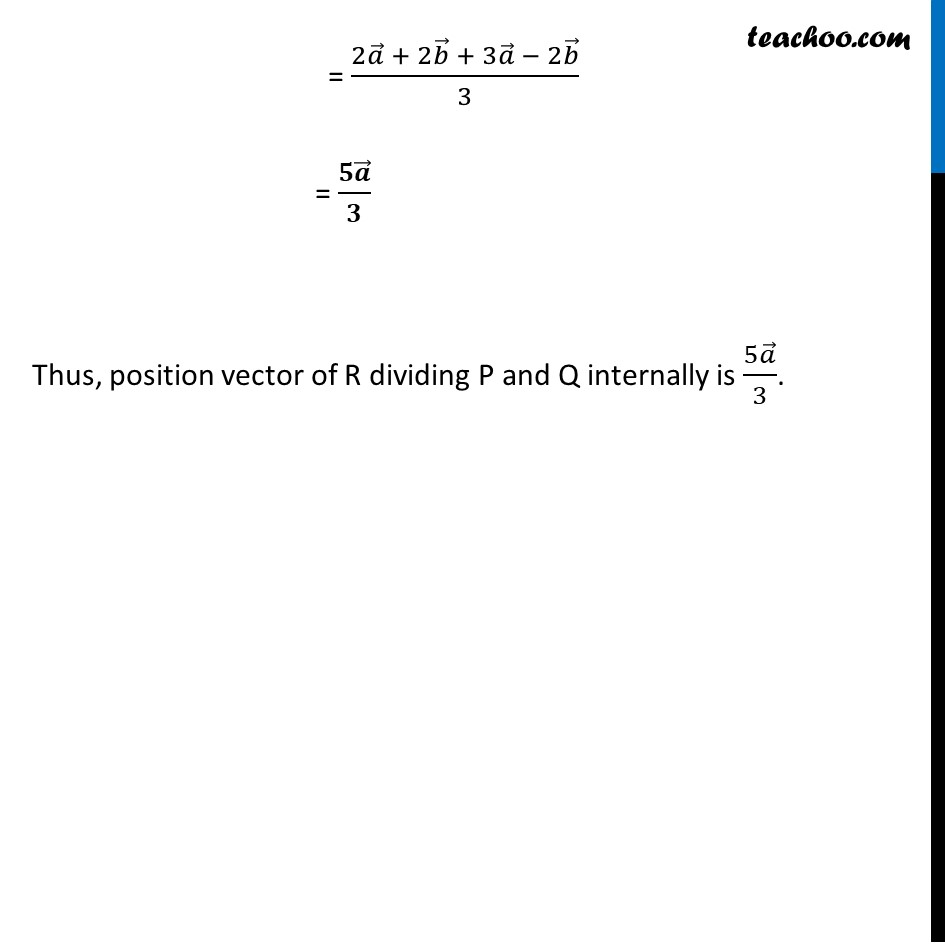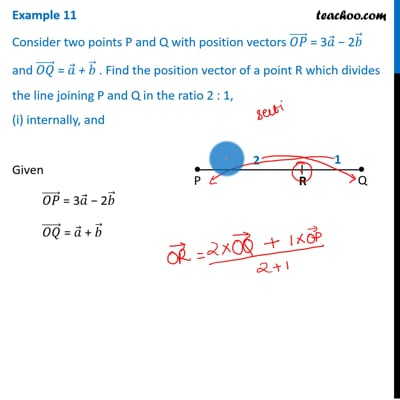Examples

Chapter 10 Class 12 Vector Algebra
Serial order wiseThis video is only available for Teachoo black users

Learn in your speed, with individual attention - Teachoo Maths 1-on-1 Class

### Transcript

Example 11 Consider two points P and Q with position vectors (𝑂𝑃) ⃗ = 3𝑎 ⃗ − 2𝑏 ⃗ and (𝑂𝑄) ⃗ = 𝑎 ⃗ + 𝑏 ⃗ . Find the position vector of a point R which divides the line joining P and Q in the ratio 2 : 1, (i) internally, and Given (𝑂𝑃) ⃗ = 3𝑎 ⃗ − 2𝑏 ⃗ (𝑂𝑄) ⃗ = 𝑎 ⃗ + 𝑏 ⃗ Position vector of R = (𝟐(𝑶𝑸) ⃗ + 𝟏(𝑶𝑷) ⃗)/(𝟐 + 𝟏) (𝑂𝑅) ⃗ = (2(𝑎 ⃗ + 𝑏 ⃗ ) + 1(3𝑎 ⃗ − 2𝑏 ⃗ ))/(2 + 1) = (2𝑎 ⃗ + 2𝑏 ⃗ + 3𝑎 ⃗ − 2𝑏 ⃗)/3 = (𝟓𝒂 ⃗)/𝟑 Thus, position vector of R dividing P and Q internally is (5𝑎 ⃗)/3.